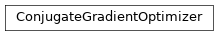# garage.torch.optimizers.conjugate_gradient_optimizer¶

Computes the decent direction using the conjugate gradient method, and then computes the optimal step size that will satisfy the KL divergence constraint. Finally, it performs a backtracking line search to optimize the objective.

class ConjugateGradientOptimizer(params, max_constraint_value, cg_iters=10, max_backtracks=15, backtrack_ratio=0.8, hvp_reg_coeff=1e-05, accept_violation=False)

Bases: torch.optim.OptimizerPerforms constrained optimization via backtracking line search.

The search direction is computed using a conjugate gradient algorithm, which gives x = A^{-1}g, where A is a second order approximation of the constraint and g is the gradient of the loss function.

Parameters
• params (iterable) – Iterable of parameters to optimize.

• max_constraint_value (float) – Maximum constraint value.

• cg_iters (int) – The number of CG iterations used to calculate A^-1 g

• max_backtracks (int) – Max number of iterations for backtrack linesearch.

• backtrack_ratio (float) – backtrack ratio for backtracking line search.

• hvp_reg_coeff (float) – A small value so that A -> A + reg*I. It is used by Hessian Vector Product calculation.

• accept_violation (bool) – whether to accept the descent step if it violates the line search condition after exhausting all backtracking budgets.

step(self, f_loss, f_constraint)

Take an optimization step.

Parameters
• f_loss (callable) – Function to compute the loss.

• f_constraint (callable) – Function to compute the constraint value.

property state(self)

dict: The hyper-parameters of the optimizer.